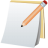##Display the time passed in any format you want

If you use digg you probably noticed that they displayed the time passed since a comment has been posted in the following way:To obtain a rezult in this format we will use the function strtotime. This function counts the seconds that have passed since the 1st of January 1970.
In order to obtain the number of seconds exactly since a comment has been posted we need to save the date for each comment in the following format: date(‘Y-m-d H:i:s’) . This will return 2008-11-12 14:39:53.

So when you create the date field in the MySQL table choose as type datetime.

Let’s start off with inserting a comment in the database.

INSERT INTO `comments` (`name` , `comment_text`, `date`) VALUES (‘Sava Stefan’, ‘This is a test comment’, NOW( ));

NOW() will insert the current date and time at the exact moment the query is executed. Eg: 2008-11-12 14:39:53.

It’s now time to show the time passed since a comment has been posted.

Now let’s create a function called countTime.

function countTime(\$comment_date) { \$now = date(‘Y-m-d H:i:s’); \$number_of_seconds = (strtotime(\$now) – strtotime(\$comment_date)); }

Until now I managed to get the number of seconds passed since the comment has been posted (\$comment_date). We go on further and obtain the number of hours and minutes with simple mathematics:

\$number_of_minutes = \$number_of_seconds / (60); \$number_of_hours = \$number_of_minutes / (60); \$number_of_hours_to_display = intval(\$number_of_hours); \$number_of_minutes_to_display = intval(\$number_of_minutes – (\$number_of_hours_to_display * 60));

As you can see the number of minutes passed is equal to the number of seconds obtained earlier (that first diference – \$number_of_seconds ) split to 60. The number of hours will also be equal to the number of minutes split to 60 as well.
I used intval to obtain the integer value so we don’t display anything like: 1,523 hours Let’s not forget to get the integer for the number of seconds as well:

\$number_of_seconds_to_display= intval(\$number_of_seconds – (\$number_of_minutes_to_display * 60 * 60) – (\$number_of_minutes_to_display * 60));

In case you don’t get this last line try looking at the clock and notice how many seconds an hour and a minute have. Let’s customize the output of the function:

echo ‘This comment was posted ‘.\$number_of_hours_to_display.’ hours, ‘.\$number_of_minutes_to_display.’ minutes and ‘.\$number_of_seconds_to_display.’ seconds ago.’;

### The complete function:

function countTime(\$comment_date) {
\$now = date(‘Y-m-d H:i:s’);
\$number_of_seconds = (strtotime(\$now) – strtotime(\$comment_date));
\$number_of_minutes = \$number_of_seconds / (60);
\$number_of_hours = \$number_of_minutes / (60);
\$number_of_hours_to_display = intval(\$number_of_hours);
\$number_of_minutes_to_display = intval(\$number_of_minutes – (\$number_of_hours_to_display * 60));
\$number_of_seconds_to_display= intval(\$number_of_seconds – (\$number_of_minutes_to_display * 60 * 60) – (\$number_of_minutes_to_display * 60));
echo ‘This comment was posted ‘.\$number_of_hours_to_display.’ hours, ‘.\$number_of_minutes_to_display.’ minutes and ‘.\$number_of_seconds_to_display.’ seconds ago.’;
}

Integrating the function is simple as hell. If you obtained your comments as an array let’s say that \$comment[‘date’] is the date value of a comment from your database.

Name: \$comment[‘name’];
Comment: \$comment[‘comment_text’];
countTime(\$comment[‘date’]);

It will display the following considering the database insert from above:

Name: Sava Stefan
Comment: This is a test comment
This comment was posted 3 hours, 24 minutes and 13 seconds ago.

Of course you can calculate the time left until a certain thing should happen:

\$number_of_seconds_until = (strtotime(\$data_in_future) – strtotime(\$now));

Get going … you time machine 😉

### Stay Connected